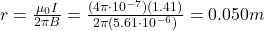## A current of 1.41 A in a long, straight wire produces a magnetic field of 5.61 uT at a certain distance from the wire. Find this dista

Question

A current of 1.41 A in a long, straight wire produces a magnetic field of 5.61 uT at a certain distance from the wire. Find
this distance.

in progress 0
6 months 2021-09-03T17:53:51+00:00 1 Answers 0 views 0

0.050 m

Explanation:

The strength of the magnetic field produced by a current-carrying wire is given by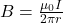where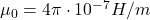is the vacuum permeability

I is the current in the wire

r is the distance from the wire

And the magnetic field around the wire forms concentric circles, and it is tangential to the circles.

In this problem, we have: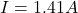(current in the wire)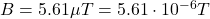(strength of magnetic field)

Solving  for r, we find the distance  from the wire: